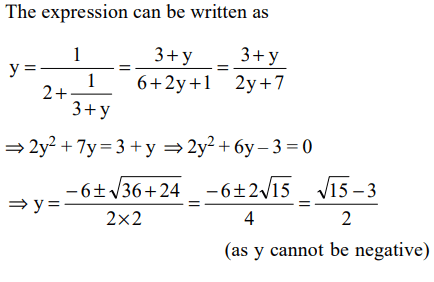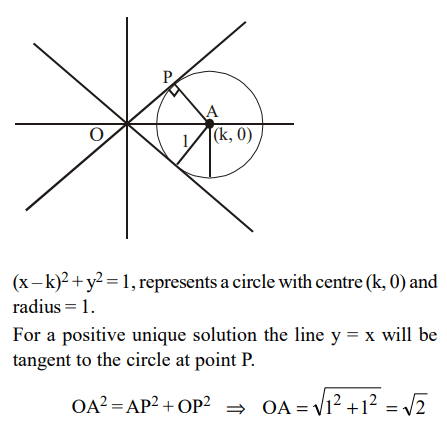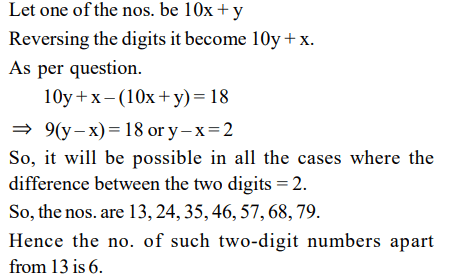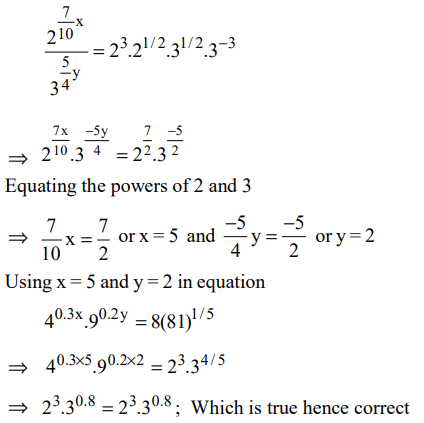## Algebra Questions and Answers Part-6

1. Let $y=\frac{1}{2+\frac{1}{3+\frac{1}{2+\frac{1}{3+.....}}}}$
What is the value of y?
a) $\frac{\sqrt{13}+3}{2}$
b) $\frac{\sqrt{13}-3}{2}$
c) $\frac{\sqrt{15}+3}{2}$
d) $\frac{\sqrt{15}-3}{2}$

Explanation:2. For which value of k does the following pair of equations yield a unique solution for x such that the solution is positive?
$x^{2}-y^{2}=0$
$\left(x-k\right)^{2}+y^{2}=1$
a) 2
b) 0
c) $\sqrt{2}$
d) $-\sqrt{2}$

Explanation:3. let $x=\sqrt{4+\sqrt{4-\sqrt{4+\sqrt{4-.......infinity }}}}$
Then x equals
a) 3
b) $\left(\frac{\sqrt{13}-1}{2}\right)$
c) $\left(\frac{\sqrt{13}+1}{2}\right)$
d) $\sqrt{13}$

Explanation:4. A telecom service provider engages male and female operators for answering 1000 calls per day. A male operator can handle 40 calls per day whereas a female operator can handle 50 calls per day. The male and the female operators get a fixed wages of Rs. 250 and Rs. 300 per day respectively. In addition, a male operator gets Rs. 15 per call he answers and a female operator gets Rs. 10 per call she answer. To minimize the total cost, how many male operators should the service provider employ assuming he has to employ more than 7 of the 12 female operators available for the job?
a) 15
b) 14
c) 12
d) 10

Explanation:5. When you reverse the digits of the number 13, the number increases by 18. How many other two-digit numbers increase by 18 when their digits are reversed?
a) 5
b) 6
c) 7
d) 8

Explanation:6. What values of x satisfy $x^{2/3}+x^{1/3}-2\leq 0$
a) $-8\leq x\leq1$
b) $-1\leq x\leq8$
c) 1 < x < 8
d) $1\leq x\leq8$

Explanation:7. What are the values of x and y that satisfy both the equations?
$2^{0.7x}.3^{-1.25y}=8\frac{\sqrt{6}}{27}$
$4^{0.3x}.9^{0.2y}=8.\left(81\right)^{1/5}$
a) x = 2, y = 5
b) x = 2.5, y = 6
c) x = 3, y = 5
d) x = 5, y = 2

Explanation:8. The number of solutions of the equation 2x + y = 40 where both x and y are positive integers and $x\leq y$
a) 13
b) 14
c) 18
d) 20

Explanation:9. The sum of four consecutive two-digit odd numbers, when divided by 10, becomes a perfect square. Which of the following can possibly be one of these four numbers?
a) 21
b) 25
c) 41
d) 6710. The price of Darjeeling tea (in rupees per kilogram) is 100 + 0.10n, on the $n^{th}$ day of 2007 (n = 1, 2, ..., 100), and then remains constant. On the other hand, the price of Ooty tea (in rupees per kilogram) is 89 + 0.15n, on the $n^{th}$ day of 2007 (n = 1, 2, ..., 365). On which date in 2007 will the prices of these two varieties of tea be equal?